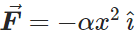# In an experiment, one of the forces exerted on a proton is F =−αx2ı^ where α=12N/m2

In an experiment, one of the forces exerted on a proton iswhere α=12N/m2.

a) How much work does F do when the proton moves along the straight-line path from the point (0.10 m, 0) to the point (0.10 m, 0.40 m)?
b) Along the straight-line path from the point (0.10 m, 0) to the point (0.30 m, 0)?
c) Along the straight-line path from the point (0.30 m, 0) to the point (0.10 m, 0)?
d) Is the force F conservative? Explain. If F is conservative, what is the potential energy function for it? Let U = 0 when x = 0.